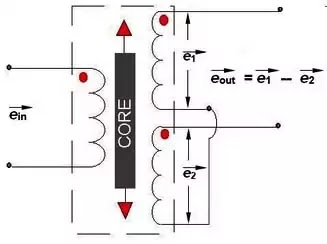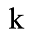## \$type=carousel\$clm=4

LVDT is Electromechanical type Inductive Transducer that converts rectilinear displacement into the Electrical Signal. LVDT is a secondary transducer.

## Introduction of LVDT

LVDT full form is Linear Variable Differential Transformer. As the name suggests, many people get confused that it is a Transformer. But actually, it is a Transducer not a Transformer. Because its working principle is same as Transformer (i.e. Mutual Induction Principle) and also the output across its secondary coil is in the form of differential voltage, that’s why it is named as Linear Variable Differential Transformer (LVDT).

It is categorised as an Inductive Transducer used to measure the speed or position of an object. Generally most Inductive Sensors work on the principle of Transformer.

Now let’s discuss the complete concepts of LVDT in detail.

## What is LVDT?

Linear Variable Differential Transformer (LVDT) is an Electromechanical type Inductive Transducer that converts rectilinear displacement into the AC Electrical Signal.

Since LVDT is a secondary transducer, hence physical quantities such as Force, Weight, Tension, Pressure, etc are first converted into displacement by a primary transducer and then LVDT is used to measure it in terms of the corresponding Electrical signal.

Since LVDT is an AC-controlled device, so there is no electronics component inside it. It is the most widely used Inductive Sensor due to its high accuracy level. Its electrical output is obtained because of the difference of secondary voltages, hence it is called Differential Transformer.

## LVDT Construction

LVDT consists of one primary winding P and two secondary windings S1 & S2 mounted on a cylindrical former. Both the secondary windings (S1 & S2) has an equal number of turns and is placed identically on either side of the primary winding in such a way that the net output will be the difference of the voltage of both secondary windings.

There is a movable soft iron core placed inside the former.Construction of LVDT

Hydrogen annealing is done on the Iron core to reduce harmonics, residual voltage of the core and thus provides high sensitivity. The movable core also is laminated in order to reduce the eddy current losses. The displacement to be measured is attached to this movable soft iron core. LVDT is placed inside the stainless steel housing because it will provide electrostatic and electromagnetic shielding.

## LVDT working principle

The working principle of LVDT is based on the mutual induction principle.

When AC excitation of 5-15 Volts at a frequency of 50-400Hz is applied to the primary winding, then a magnetic field is produced. This magnetic field induces a mutual current in secondary windings. Due to this, the induced voltages in secondary windings (S1 & S2) are E1 & E2 respectively.

Since both the secondary windings are connected in series opposition, So the net output voltage will be the difference of both induced voltages (E1 & E2) in secondary windings.

Hence Differential Output of LVDT will be  E0 = E1 – E2Working of LVDT

Now according to the position of the core, there are three cases that arise.

So Let’s discuss these three cases one by one in detail.

Case 1: When the core moves towards S1 (Max Left).When the core of LVDT moves toward Secondary winding S1.

Then, in this case, the flux linkage with S1 will be more as compared to S2. This means the emf induced in S1 will be more than the induced emf in S2. Hence E1>E2 and Net differential output voltage E0 = E1 – E2 will be positive. This means the output voltage E0 will be in phase with the primary voltage.

Case 2: When the core is at Null position.

When the core is at the null position then the flux linkage with both the secondary windings will be the same.

So the induced emf (E1 & E2) in both the windings will be the same. Hence the Net differential output voltage E0 = E1 – E2 will be zero (E0 = E1 – E2 = 0). It shows that no displacement of the core.

Case 3: When the core moves towards S2 (Max Right).When the core of LVDT moves toward Secondary winding S2. Then, in this case, the flux linkage with S2 will be more as compared to S1.

It means the emf induced in S2 will be more than the induced emf in S1. Hence E2>E1 and Net differential output voltage E0 = E1 – E2 will be negative. It means the output voltage E0 will be in phase opposition (180 degrees out of phase) with the primary voltage.

From all these three cases, we can have the following conclusions:

• The direction of the movement of an object can be identified with the help of the differential output voltage of LVDT. If the output voltage E0 is positive then this means an object is moving towards the Left from the Null position.

• Similarly, If the output voltage E0 is negative then this means the object is moving towards the Right of the Null position.

• The amount or magnitude of displacement is proportional to the differential output of LVDT. The more the output voltage, the more will be the displacement of the object.

• If we take the core out of the former then the net differential of the output of LVDT will be zero.

• In fact corresponding to both the cases, whether the core is moving either Left or Right to the Null position. Then the output voltage will be increased linearly up to 5mm from the Null position and after 5 mm output E0 will be non-linear.

The Graph of variation of output with respect to its position is shown in the below figure.Output of LVDT

Following are the main advantages and disadvantages of using LVDT as a secondary Transducer.

• Smooth and Wide Range of Operation :- LVDT has a very wide range of measurement of displacement. It can measure displacement ranging from 1.25mm to 250 mm.

•  High Sensitivity:- LVDT gives high output value so that there is no need for any Amplifier circuit for the amplification process. Typically the sensitivity of LVDT is recorded as 40V/mm.

• Low Hysteresis Losses:- LVDT gives low hysteresis losses hence repeatability is excellent under all the conditions.

• Low Friction Losses:- As the core moves in a hollow Former, So there is no concept of friction losses. Hence it gives an accurate output value.

• Rugged Operation:- It can tolerate a high degree of shock and variation, especially when the core is loaded with spring.

• Low Power consumption:- LVDT consumes very low power of approx 1W during its operation.

• Direct conversion to Electrical Signal:- They convert linear displacement directly to the corresponding electrical voltage signal which are easy to process further.

• Fast dynamic Response:- Due to the absence of Friction, Its dynamic response becomes very fast to change in a core position.

[ ##eye## Transducer - working, types and applications ]

• Since LVDT is Inductive Transducer, so it is sensitive to Stray Magnetic Field. Hence an extra setup is required to protect it from Stray Magnetic Field.

• Since it is an electromagnetic device, so it also gets affected by the vibrations and temperature variation.

## Applications of LVDT

• LVDT is used to measure the physical quantities such as Force, Tension, Pressure, Weight, etc. These quantities are first converted into displacement by the use of primary transducers and then it is used to convert the displacement to the corresponding Electrical voltage signal.

• It is mostly used in industries as well as a servomechanism.

• It is also used in Industrial Automation, Aircraft. Turbine, Satellite, hydraulics, etc.

## LVDT Specifications

• Range of Measurement:  ± 0.25 mm to ± 750 mm

• Operating Temperature: (-265 to 600) degree celsius.

• Frequency Range:  50 Hz to 20 kHz.BLOGGER: 9
1.Easy to understand

2.Nice article

3.very well explained

4.very well explained

5.6.This comment has been removed by a blog administrator.

7.This comment has been removed by a blog administrator.

8.Thanks for the all information...

9.Name

Digital Electronics,1,Electrical Basics,15,Electrical Machine,4,Electrical Q & A,2,Electronics,2,Guest Post,3,Instrumentation,4,Mathematics,4,Power Factor,3,Power System,10,
ltr
item